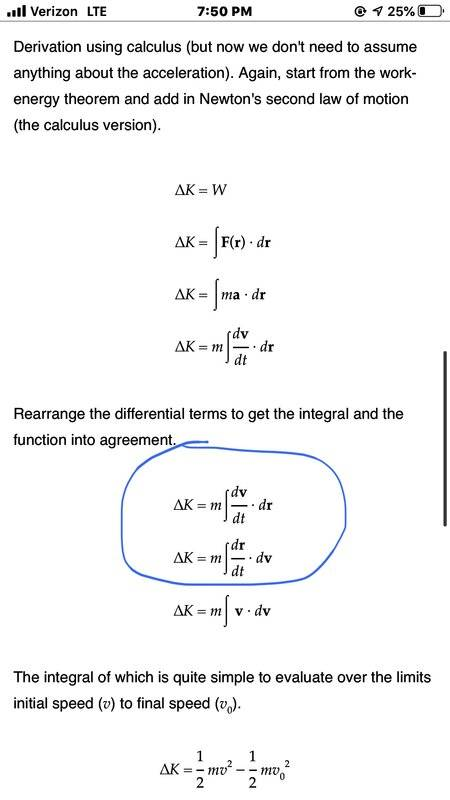# Help with deriving the formula for kinetic energy (using calculus)

EchoRush
Hello, I am learning how to use calculus to derive the formula for kinetic energy

now, I understandthe majority of the steps in how to do this, however, there is one step where I get totally lost, I will post a picture of the steps and I will circle the part where I get lost. If you see the part circled in BLUE, Why can we just “re-write” those variables? Why can we just switch the positions of the “v” and the “r”?Homework Helper
Gold Member
It might help to write ##\frac{d \mathbf v}{dt}\cdot d\mathbf r## in terms of cartesian components as $$\frac{d v_x}{dt}dx + \frac{d v_y}{dt} dy+\frac{d v_z}{dt}dz$$

Using the chain rule, $$\frac{d v_x}{dt}dx = \left(\frac{d v_x}{dx}\frac{dx}{dt}\right)dx = \frac{dx}{dt}\left(\frac{d v_x}{dx}dx\right)$$

Note $$\frac{d v_x}{dx}dx = dv_x$$

So we end up with $$\frac{d v_x}{dt}dx = \frac{dx}{dt} dv_x = v_x \, dv_x$$

Similarly for the other cartesian components.

•etotheipi, EchoRush and mpresic3
EchoRush
It might help to write ##\frac{d \mathbf v}{dt}\cdot d\mathbf r## in terms of cartesian components as $$\frac{d v_x}{dt}dx + \frac{d v_y}{dt} dy+\frac{d v_z}{dt}dz$$

Using the chain rule, $$\frac{d v_x}{dt}dx = \left(\frac{d v_x}{dx}\frac{dx}{dt}\right)dx = \frac{dx}{dt}\left(\frac{d v_x}{dx}dx\right)$$

Note $$\frac{d v_x}{dx}dx = dv_x$$

So we end up with $$\frac{d v_x}{dt}dx = \frac{dx}{dt} dv_x = v_x \, dv_x$$

Similarly for the other cartesian components.
Can you please explain what you mean when you say rewrite it in terms of Cartesian coordinates? How do you actually take (dv/dt) dr and rewrite it into Cartesian coordinates?

Homework Helper
Gold Member
Can you please explain what you mean when you say rewrite it in terms of Cartesian coordinates? How do you actually take (dv/dt) dr and rewrite it into Cartesian coordinates?

The expression ##\large \frac{d \mathbf{v}}{dt} \cdot \normalsize d\mathbf{r}## represents the dot product of two vectors.

In unit vector notation (using cartesian basis vectors ##\hat i, \hat j,## and ##\hat k##), we can write

$$\frac{d \mathbf{v}}{dt} = \frac{d{v_x}}{dt} \hat i + \frac{d{v_y}}{dt} \hat j + \frac{d{v_z}}{dt} \hat k$$
$$d \mathbf {r} = dx \hat i + dy \hat j+ dz \hat k$$

The dot product can then be expressed as
$$\frac{d \mathbf{v}}{dt} \cdot d\mathbf{r} = \frac{d{v_x}}{dt} dx + \frac{d{v_y}}{dt}dy+ \frac{d{v_z}}{dt} dz$$

Arjan82
##\mathbf{r}## is a vector pointing to the location in space of the location of ##\mathbf{v}## (where ##\mathbf{v}## is also a vector with the components of velocity). At this point you haven't decided whether you take ##\mathbf{r}## in Cartesian coordinates, meaning ##\mathbf{r} = [x, y, z]^T## or in cylindrical coordinates, meaning ##\mathbf{r} = [x, r, \theta]^T##, or spherical coordinates: ##\mathbf{r} = [r, \theta, \phi]^T##. So that is what it means to "rewrite ##\frac{d\mathbf{v}}{dt} \cdot \mathbf{dr}## into Cartesian components". It is deciding that ##\mathbf{r} = [x, y, z]^T##.

Don't forget that ##\mathbf{v}## must be described in the same coordinate system of course: ##\mathbf{v} = [v_x, v_y, v_z]^T##.

Homework Helper
Gold Member
Looking back at my post #2, I don't like what I did. I said you could use the chain rule to write ##\large \frac{dv_x}{dt} = \frac{dv_x}{dx} \frac{dx}{dt}##. This doesn't take into account that in three dimensional space ##v_x## would generally depend on all three of the coordinates ##x, y,## and ##z## and not on just ##x##.

Here's another attempt. $$\frac {d \mathbf v}{dt} \cdot d \mathbf {r} = \frac {d \mathbf {v}}{dt} \cdot \frac {d \mathbf {r}}{dt} dt = \frac {d \mathbf {r}}{dt} \cdot \frac {d \mathbf {v}}{dt}dt= \frac {d \mathbf {r}}{dt} \cdot d \mathbf {v}$$

The second step just uses the commutative property ##\mathbf{A} \cdot \mathbf{B} = \mathbf{B} \cdot \mathbf{A}## for any two vectors ##\mathbf A## and ##\mathbf B##.

•vanhees71
At this point you haven't decided whether you take ##\mathbf{r}## in Cartesian coordinates, meaning ##\mathbf{r} = [x, y, z]^T## or in cylindrical coordinates, meaning ##\mathbf{r} = [x, r, \theta]^T##, or spherical coordinates: ##\mathbf{r} = [r, \theta, \phi]^T##.

This is not correct - only in a Cartesian coordinate system are the components of the position vector with respect to the basis also the coordinates.

In a spherical coordinate system, the position vector is ##\mathbf{r} = r \mathbf{e}_r##, i.e. the representation of ##\mathbf{r}## w.r.t. the spherical tangent basis is ##[r,0,0]^T##. This is not the same as the tuple ##(r, \theta, \phi)## of spherical coordinates identifying that point in the space.

In a cylindrical coordinate system, the position vector is ##\mathbf{r} = \rho \mathbf{e}_{\rho} + z\mathbf{e}_z##, i.e. the representation of ##\mathbf{r}## w.r.t. the cylindrical tangent basis is ##[\rho, 0, z]^T##. This is not the same as the tuple ##(\rho, \phi, z)## of cylindrical coordinates identifying that point in the space.

Really, it is best to only express position vectors with respect to a constant basis in the frame, e.g. a Cartesian basis.

•Arjan82
Gold Member
I try to explain as the way keeping the terms in integral along any but fixed path.
$$\int \frac{d}{dt}v\cdot dr$$
Changing integral variable from r to t
$$\int \frac{d}{dt}v\cdot \frac{dr}{dt}dt=\int \frac{d}{dt}v\cdot v dt$$
Changing integral variable from t to v
$$\int dv \cdot v$$
Often variables r and v are not monotonous along the path ,e.g. around top ends of pendulum, we should consider it in integration.

Last edited:
Gold Member
2022 Award
It's much simpler. The work is defined as the line integral of the forces along the trajectory of the particle in motion given these forces, i.e.,
$$W=\int_{t_1}^{t_2} \mathrm{d} t \dot{\vec{x}} \cdot \vec{F}[\vec{x}(t)].$$
Now along this trajectory Newton's Law of motion holds:
$$m \ddot{\vec{x}}=\vec{F}(\vec{x}).$$
Thus the work is also given by
$$W=\int_{t_1}^{t_2} \mathrm{d} t \dot{\vec{x}} \cdot m \ddot{\vec{x}}=\int_{t_1}^{t_2} \mathrm{d} t \frac{m}{2} \mathrm{d}_t (\dot{\vec{x}}^2) = \frac{m}{2} \dot{\vec{x}}^2(t_2)-\frac{m}{2} \dot{\vec{x}}(t_1).$$
That's why it is convenient to define a new quantity called "kinetic energy",
$$E_{\text{kin}}=\frac{m}{2} \dot{\vec{x}}^2=\frac{m}{2} \vec{v}^2.$$
Then we have just derived the "work-energy theorem",
$$W=E_{\text{kin}}(t_2)-E_{\text{kin}}(t_1).$$

•TSny, Arjan82 and etotheipi